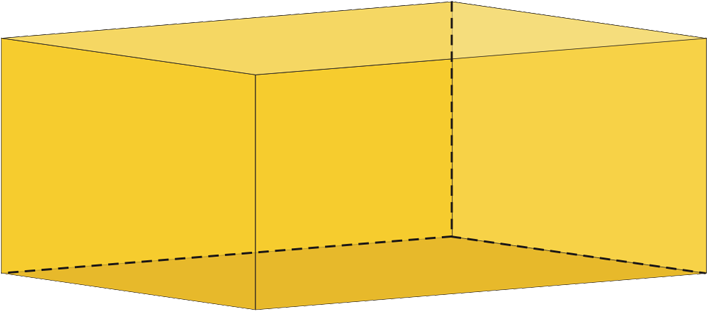# PR for Educators

Helping people for education and information# How to find volume of a rectangular prism?

The volume is the measure of how much space an object occupies. Calculate how many units of gas, air or solid can enter the space occupied by a three-dimensional object. A more technical definition is that the volume is a metric magnitude of the scalar type defined as the extension in three dimensions of a region of space.

Volume is a quantity derived from the length, since it is the result of the multiplication of three physical dimensions, such as length, height and width. Because of this, it is expressed in metric units, unlike the area magnitudes that are expressed in square units or those in length, which are expressed in linear units.

In many occasions during your studies or work, you may find yourself with the need to calculate the volume of a rectangular prism. Continue reading: How to write a conclusion for a project or an essay or a composition

## How to find volume of a rectangular prism?

Once these concepts and formulas are understood, it is very easy to calculate the volume of our prism.

• Measure the length of the prism in the system of units of your preference. The length of a rectangular prism is the measure of its longest side. Suppose we have a prism that throws a length of 15 centimeters.
• Now measure the width of the rectangular prism, which is the measure of its shortest side. We will assume as an example that our prism has a width of 6 centimeters.
• Finally, measure the height of the rectangular prism. This height is the measure that reflects how high the prism is on its vertical axis. In this example, we will say that its height is 7 centimeters.

Applying the formula V = l×a×h we would have that V = 15 cm × 6 cm × 7 cm. This would give us as a result 630 cm³.
This means that our rectangular prism occupies a volume or space equivalent to 630 cubic centimeters.

This is very easy to do and you just need to understand a few basic concepts well and apply some simple formulas. This way you can calculate the volume of a rectangular prism with great ease.### What is a rectangular prism?

A prism is a prismatoid variety that gets its name according to the shape of its base. That is, if we draw a triangle as a base we will obtain a triangular prism. If we want to draw a rectangular prism, we will use a rectangle as a base, or we will have a pentagonal prism if we use a base with five sides.

In geometry, the rectangular prism shape is described as a polyhedron whose surface is formed by two equal and parallel rectangles called bases and by four lateral faces that are also parallel rectangles and that are equal to their respective opposing faces.

Also, it receives the name of ortoedro and its better description in the eyes of a boy or of someone who initiates in the geometry would be the one of a cube that has been stretched by one of its sides a species of the cube “lengthened” up, down or back or forward.

### What is the formula for calculating the volume of a rectangular prism?

The formula for calculating the volume of a rectangle is V = (l) × ( a) × (h), where V is the Volume of the rectangular prism, while (l) is the length of the prism, (a) is the width and (h) is the height.

These measures can be expressed in any of the units of length of the systems of units accepted at an international level, such as the International System of Units (SI), the Anglo-Saxon System of Units and the Imperial System of Units.

In the International System of Units used in the USA, Latin America and a large part of Africa, the cubic meter (m3) is used as the basic unit of length, while in the Anglo-Saxon systems (used in Europe and a few countries) and in the Imperial (used in Great Britain and many of its former colonies) cubic foot and other specific measures such as the cubic yard or the cubic inch are used.